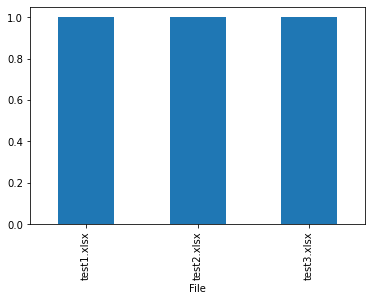# Lesson 11¶

Grab data from multiple excel files and merge them into a single dataframe.

In :
import pandas as pd
import matplotlib
import os
import sys
%matplotlib inline

In :
print('Python version ' + sys.version)
print('Pandas version ' + pd.__version__)
print('Matplotlib version ' + matplotlib.__version__)

Python version 3.7.4 (default, Aug  9 2019, 18:34:13) [MSC v.1915 64 bit (AMD64)]
Pandas version 1.0.5
Matplotlib version 3.2.2


# Create 3 excel files¶

In :
# Create DataFrame
d = {'Channel':, 'Number':}
df = pd.DataFrame(d)
df

Out:
Channel Number
0 1 255
In :
# Export to Excel

df.to_excel('test1.xlsx', sheet_name = 'test1', index = False)
df.to_excel('test2.xlsx', sheet_name = 'test2', index = False)
df.to_excel('test3.xlsx', sheet_name = 'test3', index = False)
print('Done')

Done


# Place all three Excel files into a DataFrame¶

Get a list of file names but make sure there are no other excel files present in the folder.

In :
# List to hold file names
FileNames = []

os.chdir(r"C:\notebooks\pandas")

# Find any file that ends with ".xlsx"
for files in os.listdir("."):
if files.endswith(".xlsx"):
FileNames.append(files)

FileNames

Out:
['test1.xlsx', 'test2.xlsx', 'test3.xlsx']

Create a function to process all of the excel files.

In :
def GetFile(fnombre):

# Path to excel file
location = r'C:\notebooks\pandas\\' + fnombre

# Parse the excel file
# 0 = first sheet

# Tag record to file name
df['File'] = fnombre

# Make the "File" column the index of the df
return df.set_index(['File'])


Go through each file name, create a dataframe, and add it to a list.

i.e.
df_list = [df, df, df]

In :
# Create a list of dataframes
df_list = [GetFile(fname) for fname in FileNames]
df_list

Out:
[            Channel  Number
File
test1.xlsx        1     255,
Channel  Number
File
test2.xlsx        1     255,
Channel  Number
File
test3.xlsx        1     255]
In :
# Combine all of the dataframes into one
big_df = pd.concat(df_list)
big_df

Out:
Channel Number
File
test1.xlsx 1 255
test2.xlsx 1 255
test3.xlsx 1 255
In :
big_df.dtypes

Out:
Channel    int64
Number     int64
dtype: object
In :
# Plot it!
big_df['Channel'].plot.bar();This tutorial was created by HEDARO